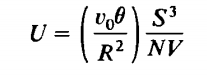### Create an Account

Already have account?

### Forgot Your Password ?

Home / Questions / Find the three equations of state for a system with the fundamental equation Corroborat...

# Find the three equations of state for a system with the fundamental equation Corroborate that the equations of state are homogeneous zero order

Find the three equations of state for a system with the fundamental equationCorroborate that the equations of state are homogeneous zero order (i.e., that T, P, and µ are intensive parameters).

Jun 09 2020 View more View Less

#### Answer (Solved)Subscribe To Get Solution